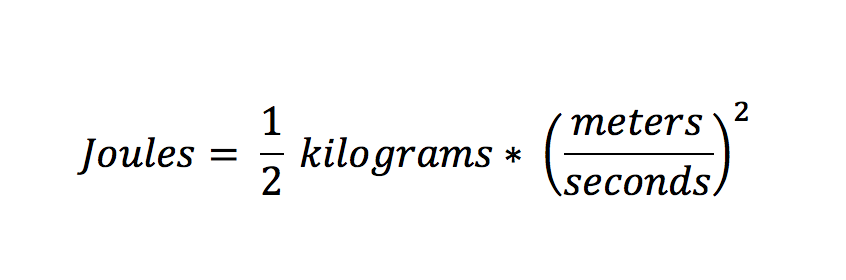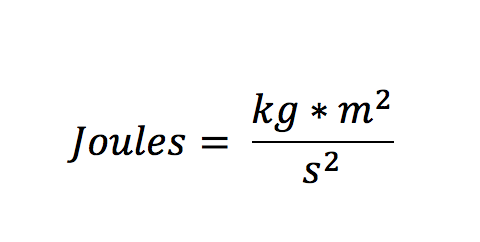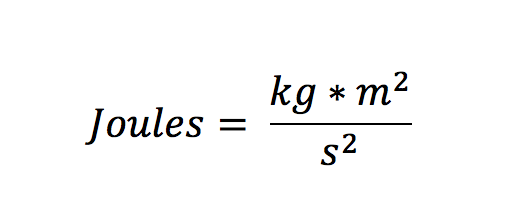# Anaheim Physics Tutor Tip: What is a Joule? A Better Understanding of EnergyA common difficulty for physics students is conceptualizing new measures and what they represent.  It’s easy enough to understand meters and seconds since we’re used to these concepts of distance and time.  Other units, however, that aren’t as common in our day-to-day can be harder to understand.  As an Anaheim physics tutor and physics teacher, I’ve found that if students have a strong understanding of units and learn to trace and connect different units, that it dramatically in both their physics understanding and their physics grades.

We have already discussed this concept when reviewing the concept of the Newton.  Now, we will be discussing another common contributor to physics confusion: the Joule.

The joule is a measure of energy.  This is probably a concept you are familiar with, but not in specific scientific terms like it is used in physics.  Here, energy is the unit that is transferred in order to do work on something or to heat something.  However, this definition is not usually very helpful in helping students understand what one joule is.  Instead, let’s try to get a better picture by looking at some of the equations that include it:Our first equation is one that you will work with often in introductory physics classes which is the equation for kinetic energy.  Here, kinetic energy is defined as one-half of the mass times the velocity squared.  This represents the energy that a moving object has.  That’s why our only variables are mass and velocity.  The heavier a moving object is, the more energy it has; and the faster an object is, the more energy it has.  Now, let’s break these variables down into their units:As we just discussed, the unit for energy (including kinetic energy) is the joule.  The unit for mass is kilograms, and velocity is meters per second.  By squaring the velocity units like in the equation, we get to this simplified unit definition of joules (note that the “one half” isn’t a variable and doesn’t have units – it’s just a number).We’ve now seen one explanation of the joule as it’s used in kinetic energy.  However, in physics, we use lots of equations for joules.  If a joule is always a unit of energy, then it should be the same regardless of which equation we use.  Let’s try again with another common introductory physics equation: potential energy.Potential energy is determined by the weight of an object and how far it is from the ground.  Specifically, this is called gravitational potential energy – it’s the energy caused from separating objects with mass from each other where there is a force from gravity.  In most introductory physics applications, the gravity we are talking about is from the earth, which is approximately 9.8 meters per seconds squared.Again, our unit of energy is the joule, mass is kilograms, the acceleration from gravity (“g”) is meters per seconds squared, and height is measured in meters.  Again, we only care about the units, so we’ll drop the 9.8 and multiply the units to get our simplified definition:Notice that we got the same answer when using both kinetic energy and potential energy: even though they’re different equations, they lead to the same definition.  Let’s try one more time with a different equation.Remember that energy can be defined as the ability to do work.  As such, we often use equations that involve equating work and energy.  So, we may have an object with a starting kinetic energy, but some of that energy is lost when it does work.  This means that work is measured in joules, but how can that be if the equation looks so different?  Work is the applied force multiplied by the distance it is applied for (specifically the displacement).  Let’s see what happens if we break it down into the units.Work is measured in joules, force is in newtons, and distance is in meters.  However, we already learned that newtons can be broken into its definition in basic units (***see here again for newton post*****).Newtons are now written as kilogram*meters per seconds squared.  This clears up our equation a lot, because now if we multiply by the last unit of meters we get our simplified equation:Which is the same as our definition that we found when using the kinetic or potential energy equations.

For fun, you can also think about the famous physics equation (that is not often used in introductory physics classes) of E=mc2.  This equation is also for energy, and is measured in joules.  Here, “c” is the speed of light, which – since it’s a speed – is measured in meters per second.  See what you get if you break this equation into its fundamental units and if it is the same as our definitions above.

Learning how these new units can be broken down into fundamental units can help with your understanding of physics and your execution of equations when taking tests or solving problems.  Take the time to break up your equations into fundamental units and you may be surprised at how related many of them are.

Book your private Anaheim physics tutor today!

Michael C. is currently a private math, science, and standardized test tutor with TutorNerds in Irvine and Anaheim.All blog entries, except for guest bloggers, are written by Tutor Nerds. Are you an education professional? If so, email us at pr@tutornerds.com for guest blogging and collaborations. We want to make this the best free education resource in SoCal, so feel free to suggest what you would like to see us write.